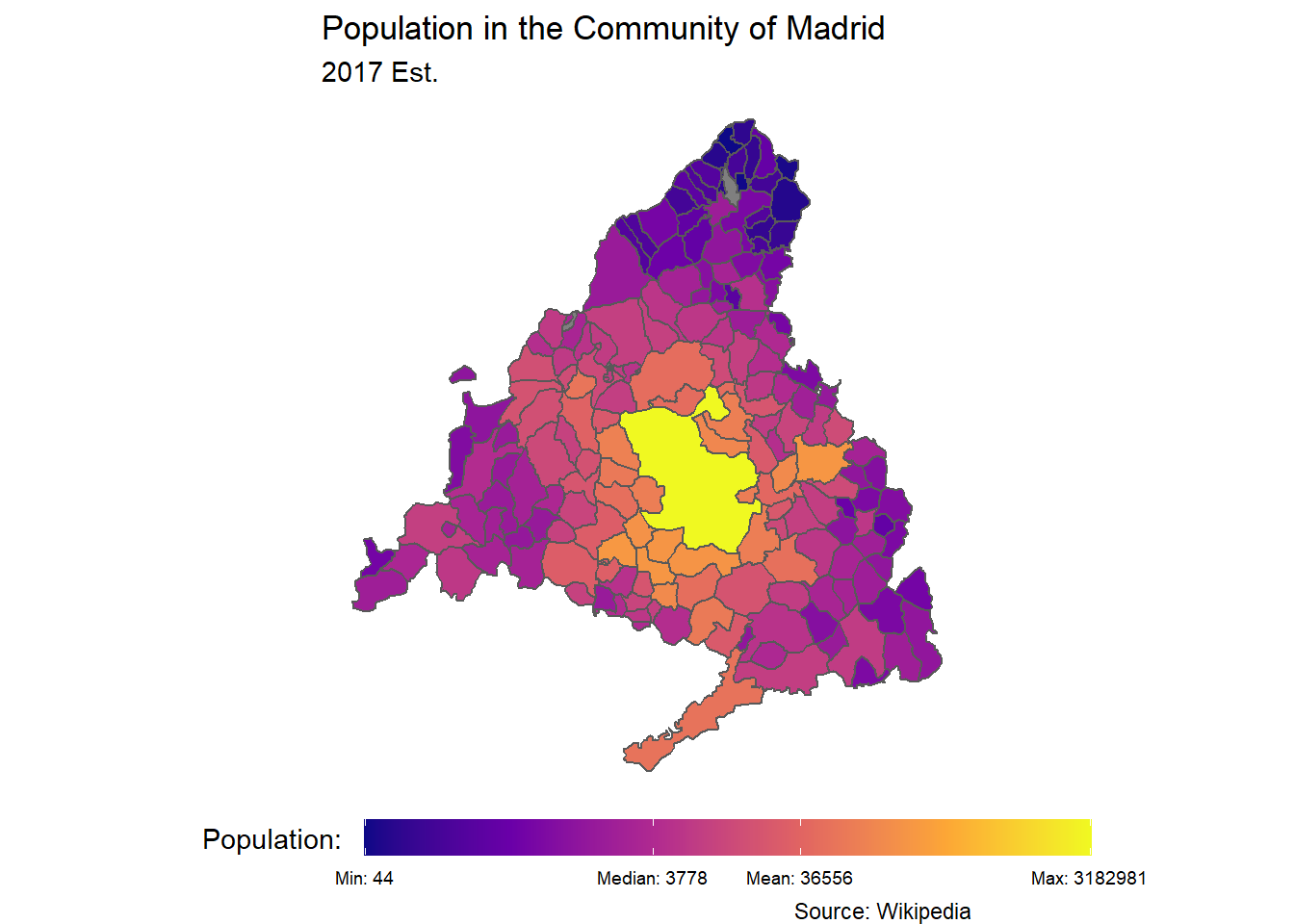# Chapter 7 Choropleth Maps

## 7.1 World Maps

The package `rnaturalearth`can handle several levels of resolution for geographic data. If you want to make your maps sharper - though a bit heavier for older machines - you can use the `scale = "medium"` or try `scale = "large"`. For the former, you should also install the package `rnaturalearthdata`.

``````library(sf)
library(rnaturalearth)``````
``world <- ne_countries(scale = "small", returnclass = "sf")``

Above, we are creating an object called `world` and getting country shapes with the command `ne_countries()`. One of the columns in this new data frame is called `geometry` which is of class sfc_MULTIPOLYGON, sfc. The package `sf` and its command `sf::geom_sf()` will use the geometry vector draw our map based on sets of coordinates.

Our `world` data frame also contains other columns with country-level information such as the country name, regional classification and even income levels.

``unique(world\$region_wb)``
``````##  "South Asia"                 "Sub-Saharan Africa"
##  "Europe & Central Asia"      "Middle East & North Africa"
##  "Latin America & Caribbean"  "Antarctica"
##  "East Asia & Pacific"        "North America"``````
``unique(world\$income_grp)``
``````##  "5. Low income"           "3. Upper middle income"
##  "4. Lower middle income"  "2. High income: nonOECD"
##  "1. High income: OECD"``````

To draw our first map, we use the familiar `ggplot2` syntax. Notice that we do not provide `x` or `y` `aes()` parameters since the these exist already in the `geometry` vector recognized by `geom_sf()`. Like in any other ggplot, we have an x and a y axis, though in this case the correspond to longitude and latitude. Knowing this, we can use other `ggplot2` commands to make our map more complex. Below we draw a horizontal line where the `yintercept` is at the equator.

``````world %>%
ggplot() +
geom_sf() +
geom_hline(yintercept = 0, linetype = "dashed") ``````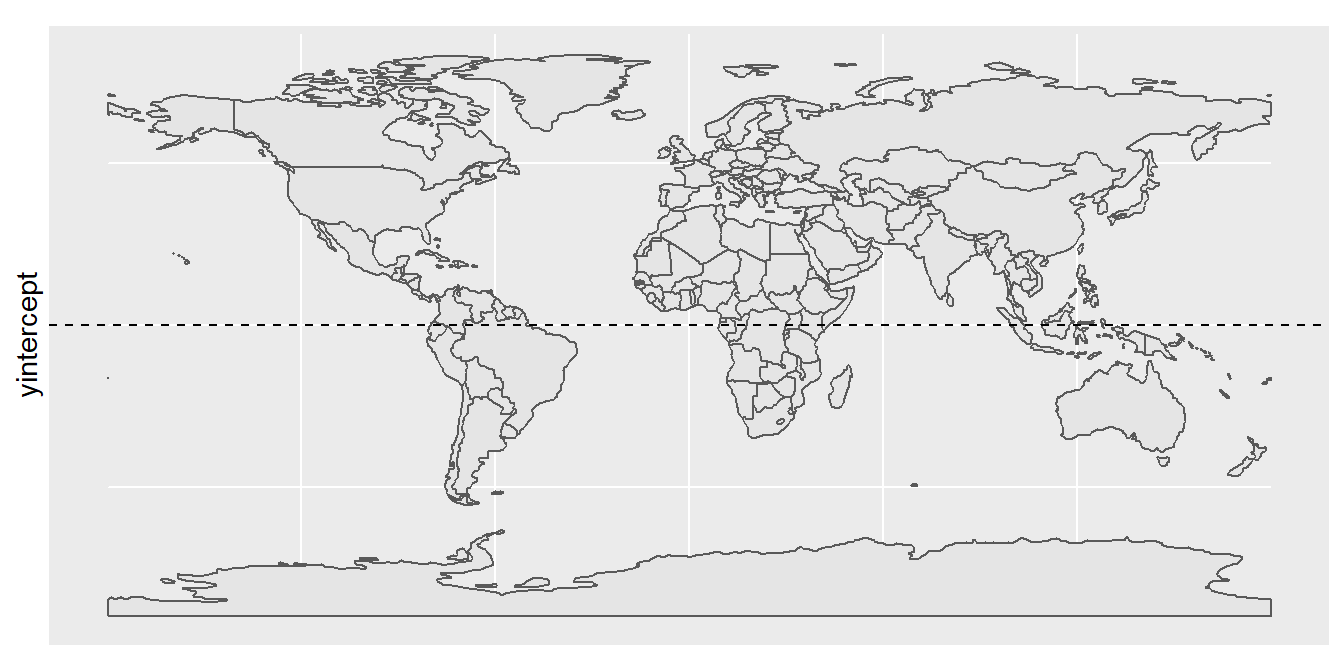Figure 7.1: Our first sf() map

``````world %>%
mutate(income_grp = factor(income_grp, ordered = T)) %>%
ggplot() +
geom_sf(aes(fill = income_grp)) +
theme_void() +
theme(legend.position = "top") +
labs(fill = "Income Group:") +
guides(fill=guide_legend(nrow=2, byrow=TRUE))``````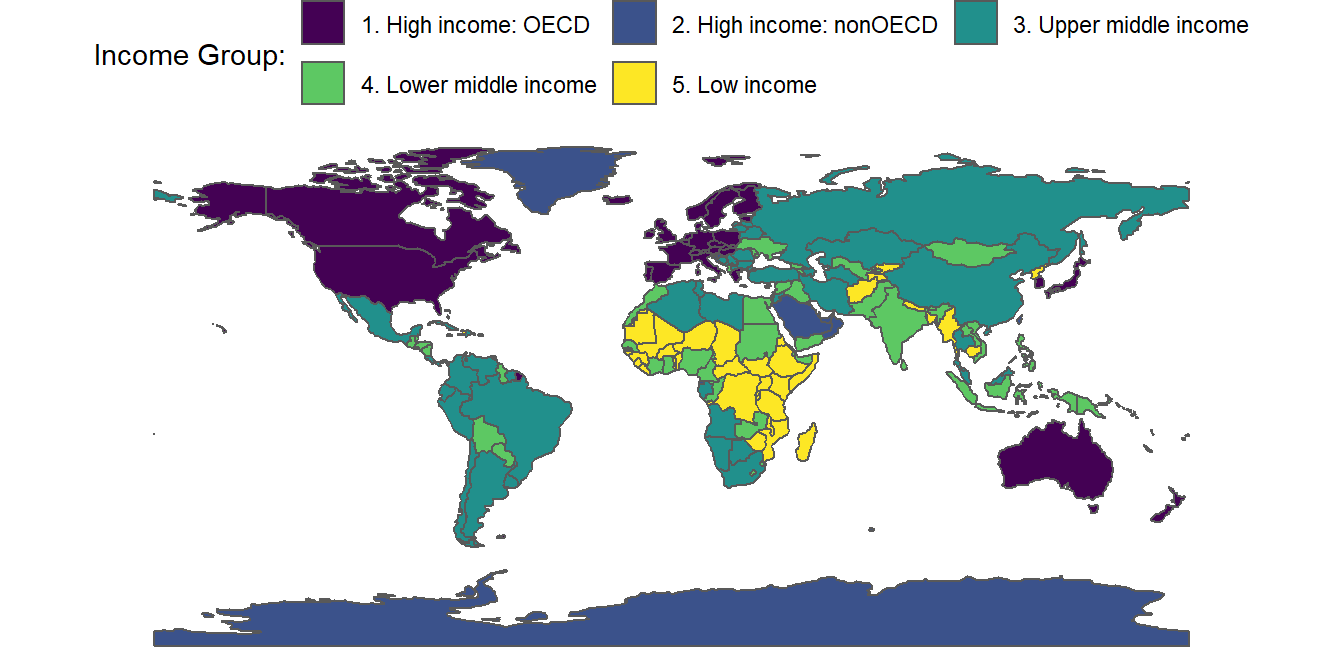Figure 7.2: Our first choropleth map

``````world %>%
ggplot() +
geom_sf(fill = NA) +
coord_sf( # Zoom-in/out
# Longitude
xlim = c(-85, -35),
# Latitude
ylim = c(10, -55)) +
theme_void()``````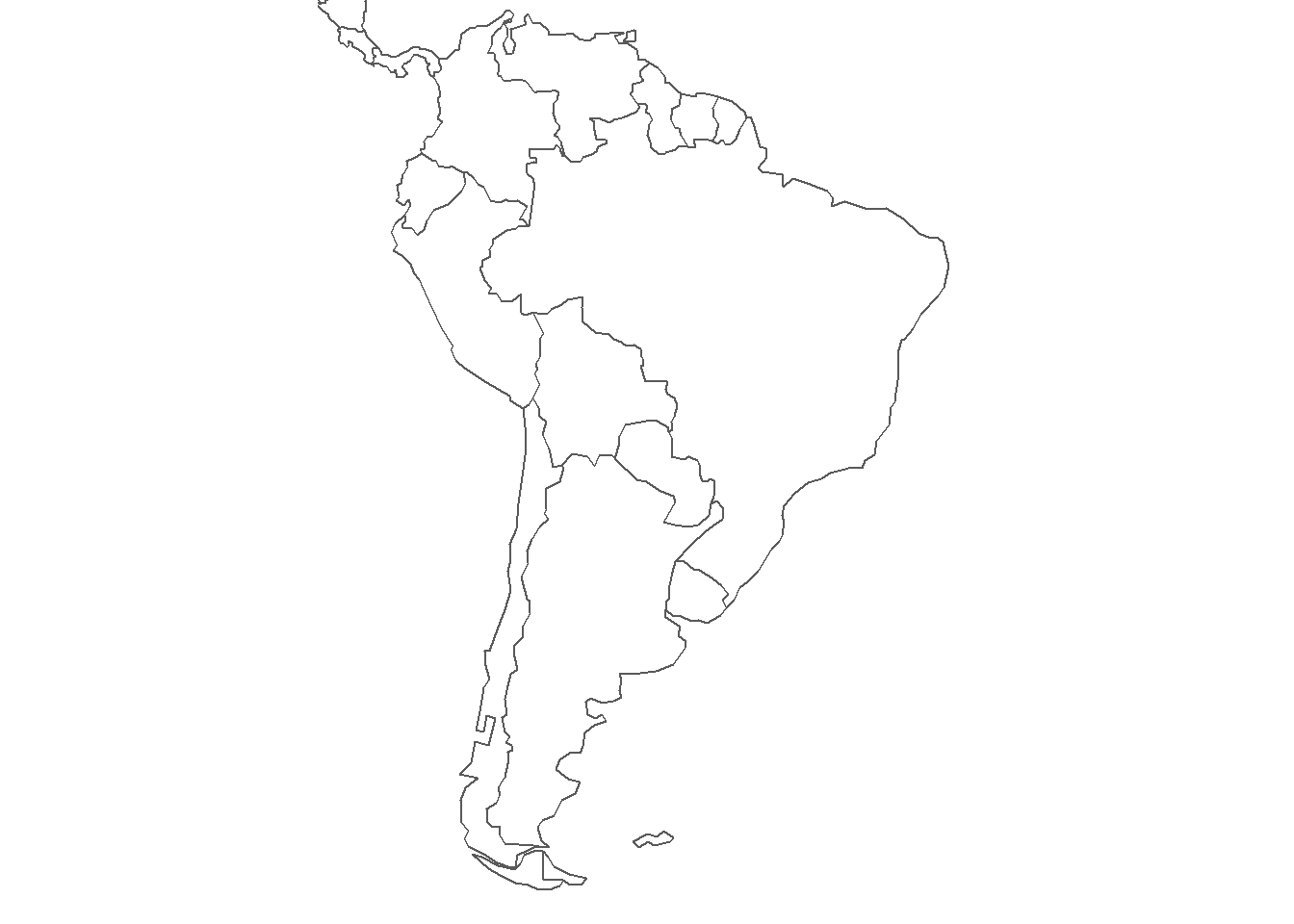Figure 7.3: Zooming-In on South America

We can add labels to our maps using the `geom_label()` or the `ggrepel::geom_label_repel()` commands to display the country names found in the vector `world\$admin`. The label requires that we define `x` and `y` coordinates, which we can get from the centroid of each country’s polygon. We obtain a rough estimate with the command `st_coordinates(st_centroid(world\$geometry))`. We can also use a high-contrast color-scale to make our choropleth maps more engaging. This is done by the command `scale_fill_viridis_c()` in the pipeline below.

``````world %>%
cbind(st_coordinates(st_centroid(world\$geometry))) %>%
"Paraguay","Uruguay","Bolivia")) %>%
mutate(pop_est = pop_est / 1000000) %>%
ggplot() +
geom_sf(data = world, fill = "gray70", color = NA) +
geom_sf(aes(fill = pop_est)) +
scale_fill_viridis_c() +
coord_sf(
xlim = c(-85, -35),
ylim = c(10, -55)) +
size = 3,
fontface = "bold") +
labs(fill = "Pop. Est. \n Millions:") +
theme_void() +
theme(plot.background = element_rect(fill = "aliceblue", color = NA))``````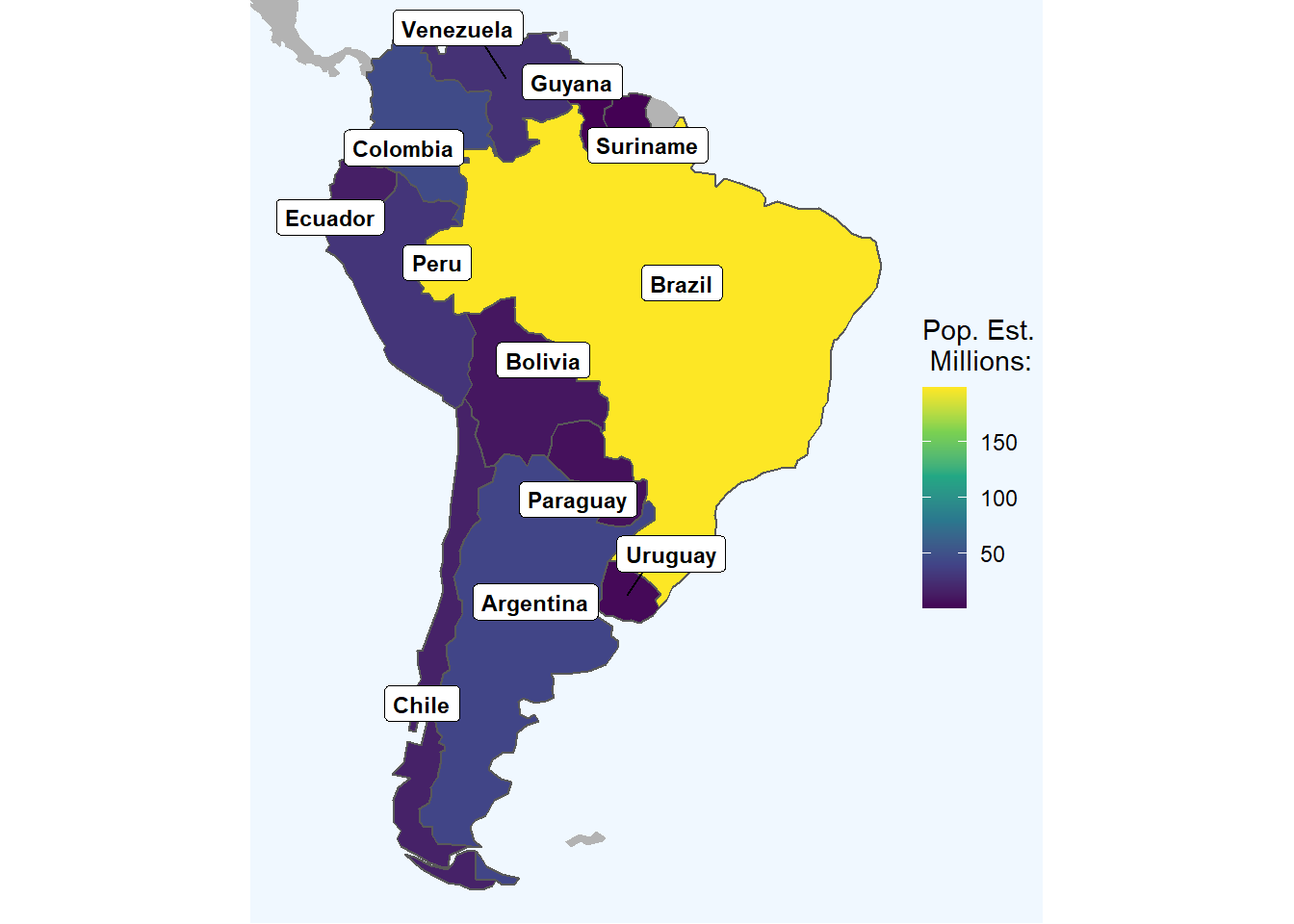Figure 7.4: Zooming-In on South America

## 7.2 Country Maps

``````spain_communities = st_read("data/ESP_adm/ESP_adm1.shp") %>%
st_transform(4326) %>%
st_as_sf()

spain_communities <- cbind(spain_communities,
st_coordinates(st_centroid(spain_communities\$geometry)))``````
``````spain_communities %>%
ggplot() +
geom_sf() +
geom_label(aes(X, Y, label = NAME_1))``````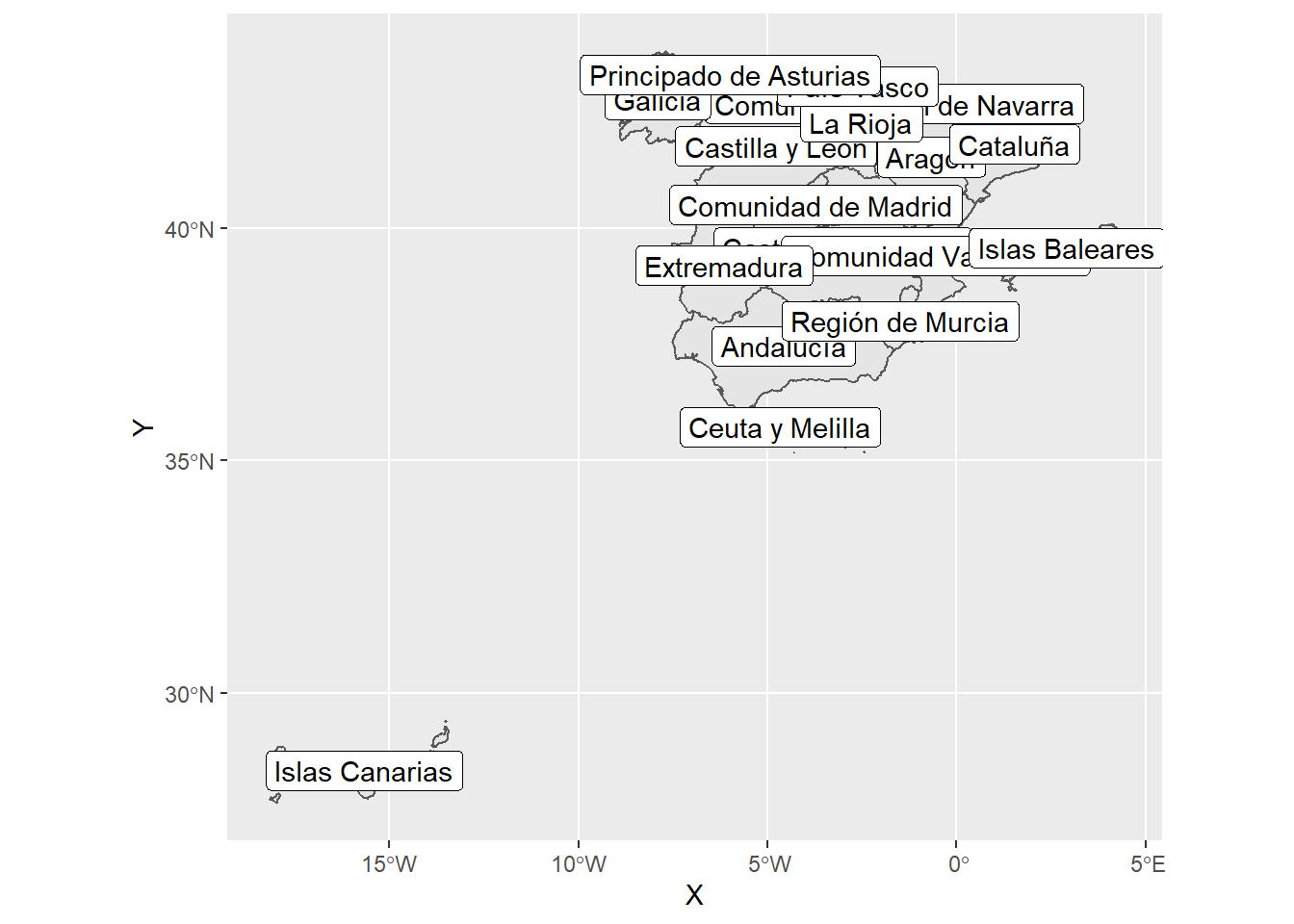``````# At the level of Provinces
st_transform(4326) %>%
st_as_sf()``````
``````## Reading layer `ESP_adm2' from data source `C:\Users\alhdz\Documents\IBEI\Data Visualization\data_viz_ir\data\ESP_adm\ESP_adm2.shp' using driver `ESRI Shapefile'
## Simple feature collection with 52 features and 11 fields
## Geometry type: MULTIPOLYGON
## Dimension:     XY
## Bounding box:  xmin: -20 ymin: 30 xmax: 4 ymax: 40
## Geodetic CRS:  WGS 84``````
``````# The Municipalities of Catalonia
st_transform(4326) %>%
st_as_sf() %>%
filter(NAME_1 == "Cataluña")``````
``````## Reading layer `ESP_adm4' from data source `C:\Users\alhdz\Documents\IBEI\Data Visualization\data_viz_ir\data\ESP_adm\ESP_adm4.shp' using driver `ESRI Shapefile'
## Simple feature collection with 8302 features and 14 fields
## Geometry type: MULTIPOLYGON
## Dimension:     XY
## Bounding box:  xmin: -20 ymin: 30 xmax: 4 ymax: 40
## Geodetic CRS:  WGS 84``````
``````spain_provinces %>%
filter(NAME_1 == "Cataluña") %>%
ggplot() +
geom_sf(aes(fill = NAME_2),
color = "black",
size = 1) +
geom_sf(data = cat_municiaplities,
fill = NA) +
geom_sf(data = filter(cat_municiaplities, NAME_4 == "Barcelona"),
fill = "black",
color = "white") +
labs(fill = "Province") +
theme_void()``````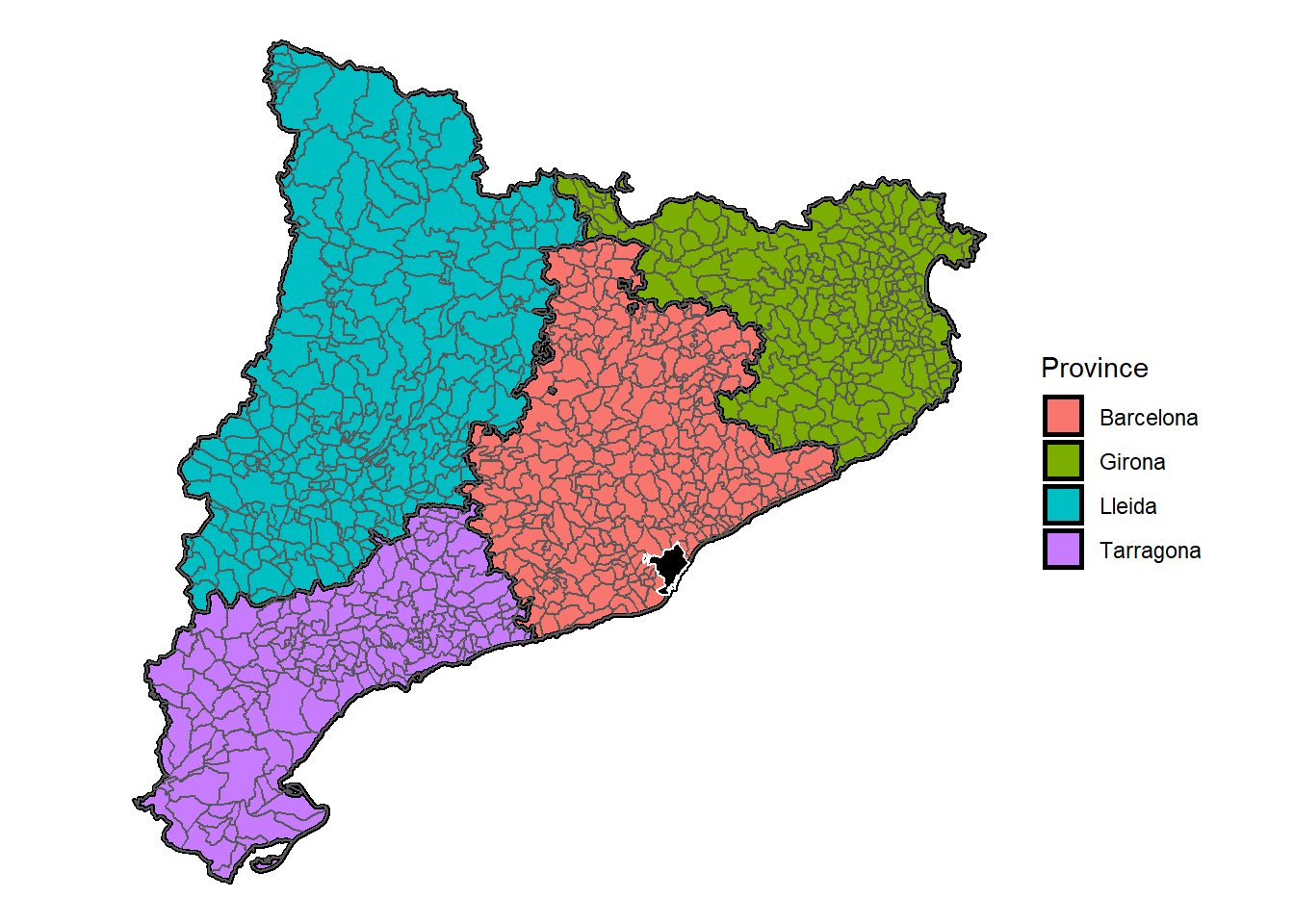## 7.3 Scraping and Maping

In Chapter 5, we went over the basics of webscraping. Here we use the same principles to obtain population data for spanish municipalities from the internet and then use them to make a map.

``````library(rvest)

# Some Data Collection
html_table() %>%
simplify() %>%
first()

# Some Data Cleaning
cat_pop <- cat_pop %>%
select(Municipality, `Population(2014)`) %>%
rename(NAME_4 = Municipality,
Population = `Population(2014)`) %>%
mutate(Population = str_remove_all(Population, "[:punct:]"),
Population = as.numeric(Population))

# Merging Data
cat_municiaplities = left_join(cat_municiaplities, cat_pop)``````
``````# A Choropleth  Map
cat_municiaplities %>%
ggplot() +
geom_sf(aes(fill = Population)) +
scale_fill_viridis_c(option = "plasma", # try "magma"
trans = "log", # try "sqrt"
breaks=c(min(cat_municiaplities\$Population, na.rm = T),
median(cat_municiaplities\$Population, na.rm = T),
mean(cat_municiaplities\$Population, na.rm = T),
max(cat_municiaplities\$Population, na.rm = T)),
labels=c(sprintf("Min: %s",min(cat_municiaplities\$Population, na.rm = T)),
sprintf("Median: %s", median(cat_municiaplities\$Population, na.rm = T)),
sprintf("Mean: %s", mean(cat_municiaplities\$Population, na.rm = T) %>%
round(digits = 0)),
sprintf("Max: %s", max(cat_municiaplities\$Population, na.rm = T)))
) +
labs(fill = "Population: \n",
caption = "Source: Wikipedia") +
theme_void() +
theme(legend.position = "bottom",
legend.key.width= unit(1, "in"), # imperial metrics
legend.key.height = unit(.3, "cm"), # or normal
plot.background = element_rect(fill = "white", color = NA))``````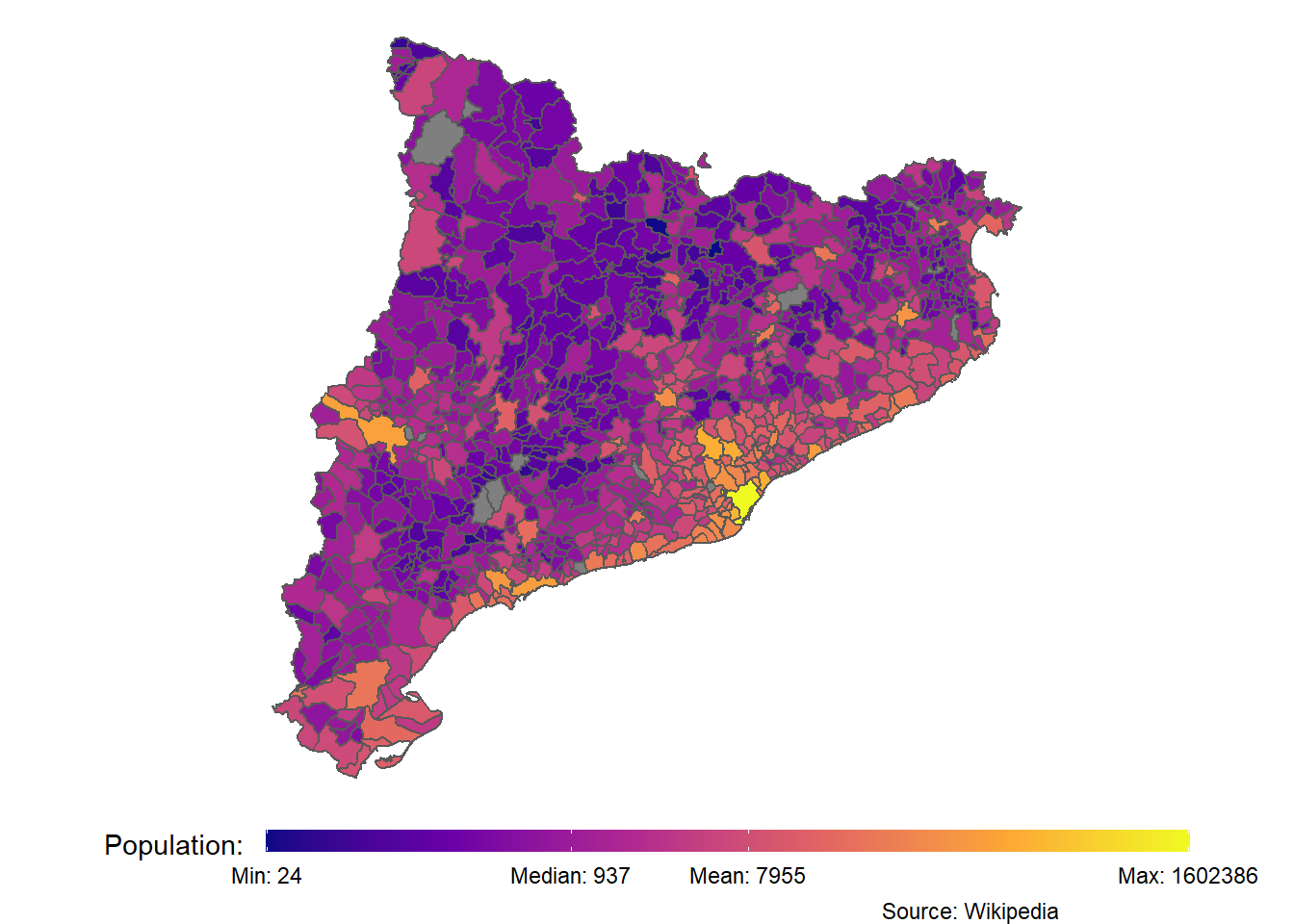## 7.4 Challenge

This link has data on the municipalities of the Community of Madrid. Can you make a population map like the one below?